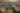# Amortized variance reduction for doubly stochastic objectives

Date March 9, 2020
Authors Ayman Boustati, Sattar Vakili, James Hensman, ST John

Approximate inference in complex probabilistic models such as deep Gaussian processes requires the optimisation of doubly stochastic objective functions. These objectives incorporate randomness both from mini-batch subsampling of the data and from Monte Carlo estimation of expectations. If the gradient variance is high, the stochastic optimisation problem becomes difficult with a slow rate of convergence. Control variates can be used to reduce the variance, but past approaches do not take into account how mini-batch stochasticity affects sampling stochasticity, resulting in sub-optimal variance reduction. We propose a new approach in which we use a recognition network to cheaply approximate the optimal control variate for each mini-batch, with no additional model gradient computations. We illustrate the properties of this proposal and test its performance on logistic regression and deep Gaussian processes.

View the paper

Share
,,